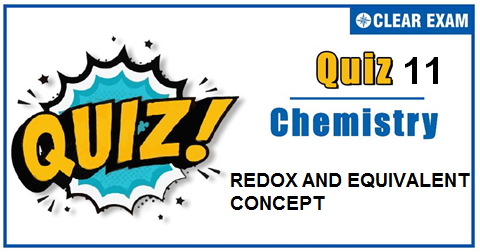## [LATEST]\$type=sticky\$show=home\$rm=0\$va=0\$count=4\$va=0

The one subject in NEET which is candidates who can easily attain good marks is Chemistry. That's the reason, often, one doesn't pay notice and choose to compromise it. But if one wants to rank above others, the tip is to be thorough with NEET chemistry concepts. The understanding of reactions and definite basic understanding is what requires major attention in Chemistry but once done it only gets simpler from there. The main focus on the to-do list should be on getting a hang of the NCERT syllabus of NEET chemistry. .

Q1. Nitrogen has fractional oxidation number in
•  N2 H4
•  NH4
•  HN3
•  N2 F2
Solution
3 × a+1 × 1=0 ∴a= -1/3

Q2.Which of the following is a redox reaction?
•  NaCl+KNO3⟶NaNO3+KCl
•  CaC2 O4+2HCl⟶CaCl2+H2 C2 O4
•  Ca(OH)2+2NH4 Cl⟶CaCl2+2NH3+2H2 O
•  2K[Ag(CN)2]+Zn⟶2Ag+K2 [Zn(CN)4]
Solution
2K[Ag(CN)2 ]+Zn→2Ag+K2 [Zn(CN)4] K2 [Zn(CN)4

Q3.  What volume of O2 measured at standard conditions will be formed by the action of 100 mL of 0.5 N KMnO4 on hydrogen peroxide in an acidic solution? The skeleton equation for the reaction is, KMnO4+H2 SO4+H22 ⟶KHSO4+MnSO4+H2 O+O2
•   0.12 litre
•  0.28 litre
•  0.56 litre
•  1.12 litre
Solution
Meq.of O2=Meq.of KMnO4=100 × 0.5 w/8 × 1000=50 ∴w(O2)=0.4 g ∴V(O2 )=(224 × 0.4)/32=0.28 litre

Q4. For redox reaction,MnO4-+C2O4(2-)+H+⟶Mn(2+)+CO2+H2 O coefficient of reactants in balanced states are MnO4- C2 O4(2-) H+
•  2 5 16
•  16 5 2
•  5 16 2
•  2 16 5
Solution
Following is balanced redox reaction. 2MnO4-+5C2 O4(2-)+16H+⟶2Mn(2+)+10CO2+8H2 O So, coefficients of MnO4-,C2 O4(2-) and H+ are 2,5,and 16 respectively.

Q5.The oxidation state of nitrogen varies from :
•  −3 to +5
•  0 to +5
•  −3 to 1
•  +3 to +5
Solution
Minimum ox.no. = group no. −8. Maximum ox.no. = group no.

Q6. Which of the following is redox reaction?
•  N2 O5+H2 O ⟶ 2HNO3
•  AgNO3 + KI ⟶ AgI+KNO3
• BaO2+H2 SO4 ⟶ BaSO4+H2 O2
•  SnCl2 + HgCl2 ⟶ SnCl4+Hg
Solution
Sn(2+)⟶Sn(4+)+2e;2e+Hg(2+)⟶Hg0

Q7.Which of the following reaction involves oxidation and reduction?
•  NaBr+HCl⟶NaCl+HBr
•  HBr +AgNO3⟶AgBr+HNO3
•  H2+Br2⟶2HBr
•  Na2O+H2 SO4⟶Na2 SO4+H2 O
Solution
Only this reaction involves oxidation and reduction.

Q8.What volume of N K2 Cr2 O7 solution is required to oxidise (in acid solution) a solution containing 10 g of FeSO4? (mol.wt.of FeSO4= 152)
•  65.78 mL
•  T134 mL
•  35 mL
•  33.5 mL
Solution
Meq.of K2 Cr2 O7=Meq.of FeSO4 1 × V=10/(152/1) × 1000 ∴V=65.78 mL

Q9.Which of the following is not a redox reaction?
•  2Na+Cl2→2NaCl
•  C+O2→CO2
•  AgNO3+NaCl→AgCl+NaNO3
•  Zn+H2 SO4→ZnSO4+H2
Solution
None of elements in reaction (c) undergoes a change in oxidation number, therefore reaction (c) is not a redox reaction

Q10. In the standardization of Na2 S2 O3 using K2 Cr2 O7 by iodometry, the equivalent weight of K2 Cr2 O7 is :
•  (molecular weight)/2
•  (molecular weight)/6
•  (molecular weight)/3
• Same as molecular weight
Solution
Cr2(6+)+6e ⟶2Cr(3+); ∴Eq.wt.=(mol.wt.)/6## Want to know more

Please fill in the details below:

## Latest NEET Articles\$type=three\$c=3\$author=hide\$comment=hide\$rm=hide\$date=hide\$snippet=hide

Name

ltr
item
BEST NEET COACHING CENTER | BEST IIT JEE COACHING INSTITUTE | BEST NEET & IIT JEE COACHING: REDOX AND EQUIVALENT CONCEPT QUIZ-11
REDOX AND EQUIVALENT CONCEPT QUIZ-11